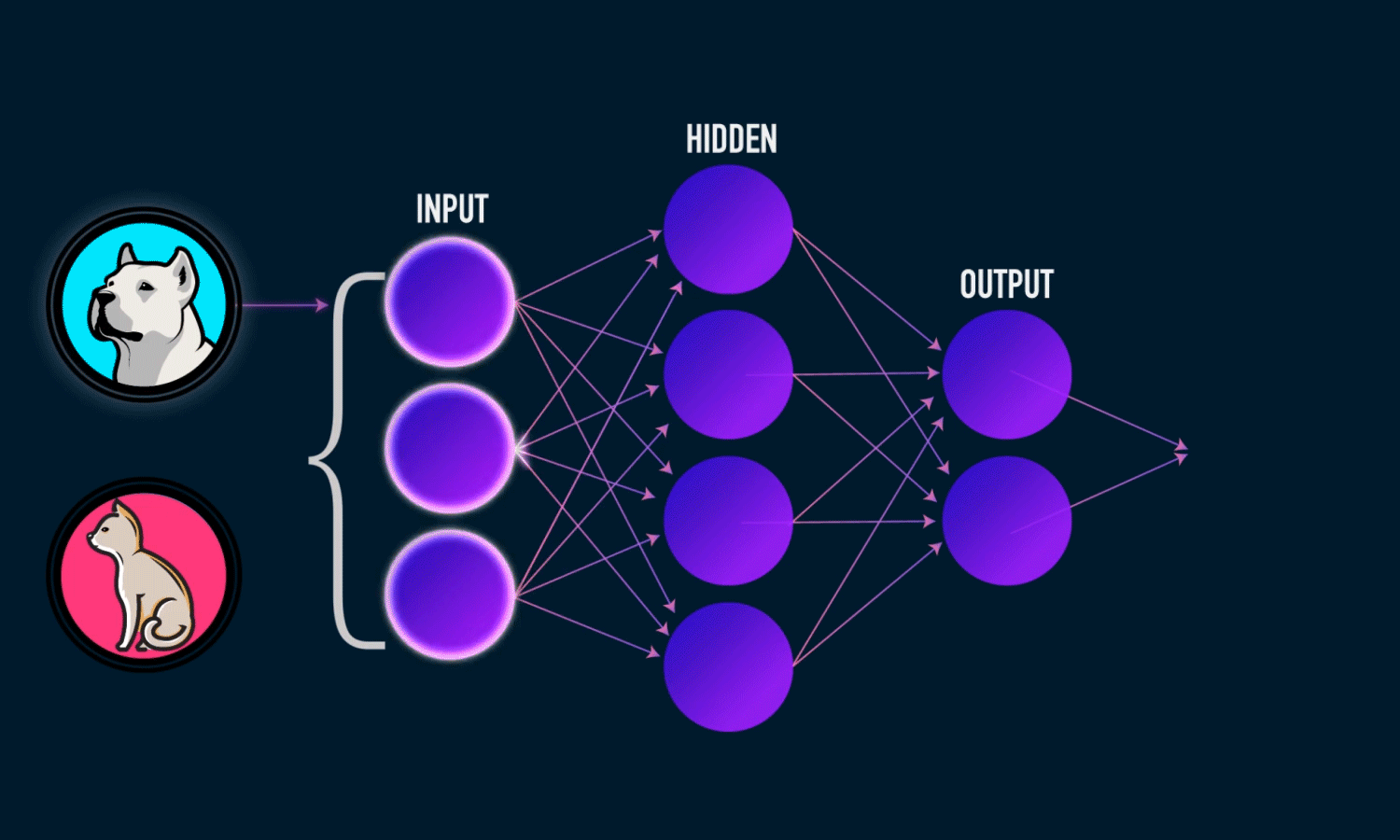Activation Functions Explained

Source: Deep Learning on Medium

Disclaimer: Readers of this article are assumed to have a basic understanding of deep learning and neural networks. If you are new to these concepts, here are some great resources to help you get started. Hope you enjoy my article :)

What is an Activation Function?

In an artificial neural network, an activation function of a neuron determines the output of that neuron based on its inputs.

Pre-output = input * weights + bias

Output = Activation(pre-output)

This result is what decides whether or not the neuron/node gets fired. I won’t go deep into the biology here, but this concept is based upon the idea that the neurons in our brain fire depending on the stimuli they receive.

There are 2 main types of activation functions: Linear and Non-Linear

Linear Functions

As seen below, a linear function (f(x) = a +bx) is a graph that is literally a straight line.

Utilizing a linear function in a hidden layer would essentially render said layer useless because the composition of 2 linear functions is in itself a linear function. (Remember the weighted sum of inputs is a linear function!). Basically, if you were to have a neural network with only linear functions, it would be the same as having a single layer perceptron.

Non-Linear Functions

Non-Linear functions as the name suggest, do not look like a straight line when graphed out. These are the most commonly used activation functions, and for good reason. Neural Networks are meant to be Universal function approximators meaning it can represent any function. Now if we want a neural network to be able to implement a very complex function, it’s unable to do so with just linear activation functions. Therefore, on a high level, the introduction of Non-Linear functions allows for a network to approximate arbitrary complex functions.

Commonly Used Activation Functions

The following are the most commonly seen functions utilized in neural networks.

1. Sigmoid Function

The sigmoid activation function can be expressed with the formula: f(x) = 1/1+exp(-x). The range of outputs for this function is between 0 and 1. A large positive input results in an output close to 1, while a large negative input results in an output close to 0. This function is often utilized for classification problems since all probabilities lie between 0 and 1. The function forms an S-shaped graph as shown below.

There are however several cons associated with using this function:

Looking towards the ends of the graph, you can see that y values start responding less to changes in x values. This means that the closer the graph gets to the horizontal asymptotes, the smaller the gradients get. Essentially, they start to “vanish”. This results in a network that is unable to update its weights and learn. This idea is closely linked to the issue of saturated neurons. Saturated neurons, in this case, would output values close to 0 or -1. These neurons tend to change their values very slowly which can a big problem when the neuron is wrong.

The output isn’t zero centered

When all the data coming into a neuron is positive, the gradients computed for the weights during backpropagation will either be all positive, or all negative. This results in undesirable zig-zag dynamics in the gradient update of the weights.

2. Tanh Function

The Tanh(Hyperbolic Tangent) function is represented with the formula: f(x) = 1- exp(-2x)/1+exp(-2x). Looking at the graph representing the function, you may notice that it looks very similar to the sigmoid function. This is because the TanH function is simply a scaled version of the sigmoid function.

This function still faces the issue of a vanishing gradient, but because its output values range from -1 to 1, the issue of the output not being centered around zero is eliminated.

3. ReLU Function

The ReLU (Rectified Linear Unit) function can be represented with the formula: f(x) = max(0,x). This simply means that all negative inputs are outputted as a 0, while positive inputs retain their value. This is currently the most commonly used activation function in deep learning models.

The pros of this activation function compared to others is that it accelerates convergence, and is less computationally expensive because it involves simpler mathematical operations. There is, however, a con uniquely associated with this function. The “death” of neurons. A neuron is considered dead when it only outputs 0 regardless of its input. Once a neuron reaches such a state, it is unlikely to ever recover.

How do you fix this problem? With the “leaky” ReLU function

The leaky ReLU function fixes the issue of dying neurons by introducing a small negative slope (approx. 0.01).